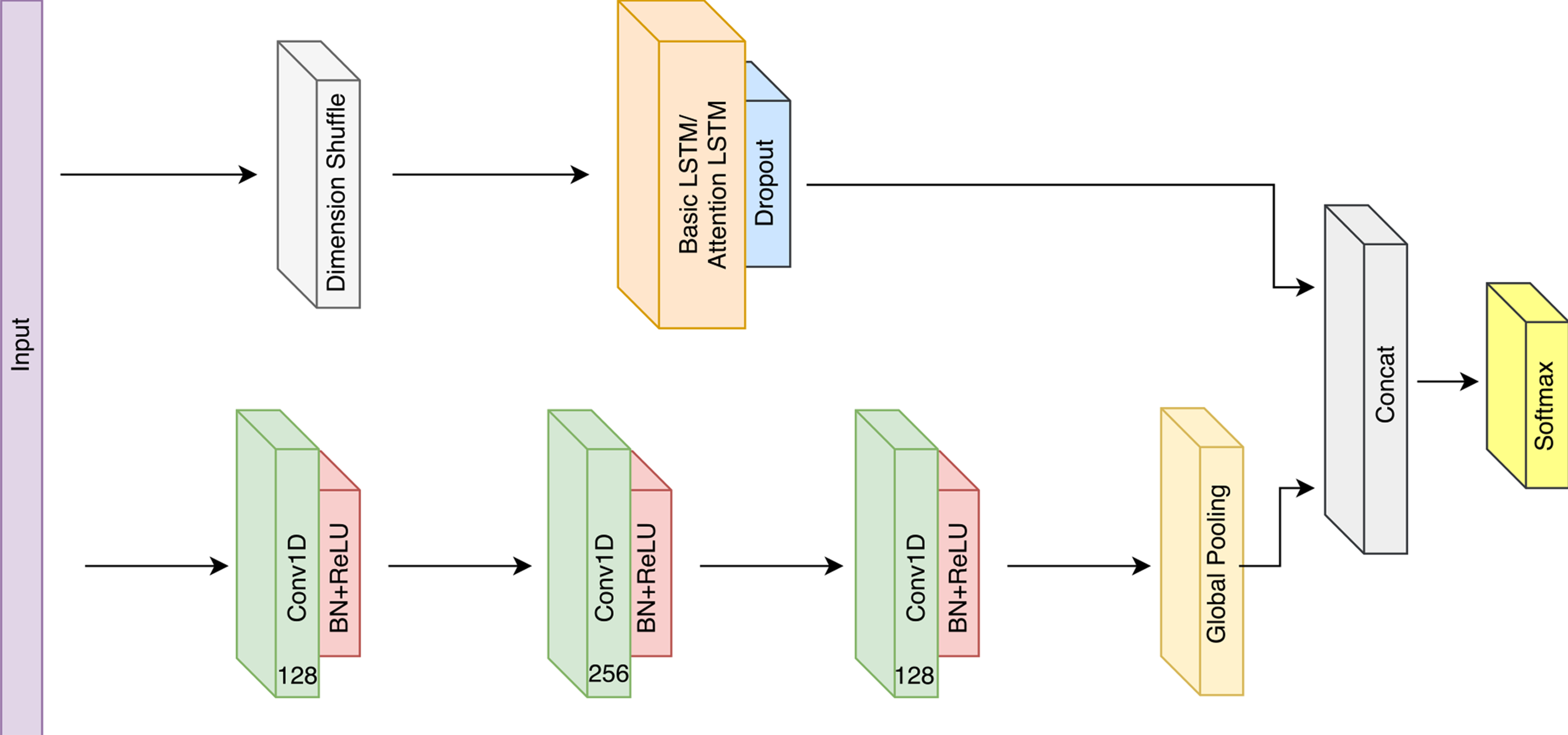# Implementing LSTM-FCN in pytorch - Part I

The follwoing article implements Multivariate LSTM-FCN architecture in pytorch. For a review of other algorithms that can be used in Timeseries classification check my previous review article.

## Network Architecture### LSTM block

The LSTM block is composed mainly of a LSTM (alternatively Attention LSTM) layer, followed by a Dropout layer.

A shuffle layer is used at the begning of this block in case the number of time steps N (sequence length of the LSTM layer), is greater than the number of variables M.

This tricks improves the efficiency as the LSTM layer since it will require M time steps to process N variables, instead of N time steps to process M variables each timestep in case no shuffle is applied.

In pytorch, the LSRM block looks like the following:

### FCN block

The core component of fully convolutional block is a convolutional block that contains:

• Convolutional layer with filter size of 128 or 256.
• Batch normalization layer with a momentum of 0.99 and epsilon of 0.001.
• A ReLU activation at the end of the block.
• An optional Squeeze and Excite block.

In pytorch, the a convolutional block looks like the following:

The fully convolutional block contains three of these convolutional blocks, used as a feature extractor. Then it uses a global average pooling layer to generate channel-wise statistics.

In pytorch, a FCN block would look like:

### LSTM-FCN

Finally, putting together the previous blocks to construct the LSTM-FCN architecture by concatenating the out of the blocks and passing it throgh a softmax activation to generate the final output.

## Training

Part II discusses the training setup of the LSTM-FCN architecture using different Datasets.